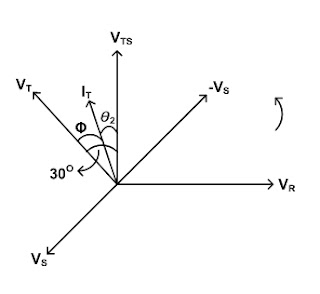### Power in Three Phase Circuit

Instrument Transformer and Power Management (P1) Course
Chapter (5) : Power Measurement in 3-ph Circuits
5.1 Power in Three Phase Circuits :
Blondel's theorem :
In any system whatsoever, of "N" wires, the true power may be measured by connecting a wattmeter in each line but one ( N-1 wattmeters), the current coil being connected in the line and the potential coil connected between that line and the line which contain no current coil. The total power for any load is the algebraic sum of the wattmeter readings. This is known as Blondel's theorem.
Applying the above theorem to any three phase, three wire circuit, true power may be measured by with tow wattmeters under all condition of loads, balance and P.F the instantaneous power is :

Pins = e1 I1 + e2 I2 + e3 I3 , but I1 + I2 + I3 = 0  then , I2 = -I1 - I3
Pins = e1 I1 + e2 ( -I1 - I3 ) + e3 I3

Wattmeter, "W1." measures the power which the current coil is IR and the voltage coil is VRS ( line voltage ) , or its power is ( e1 - e1 ) I1
Wattmeter "W2" measures the power which the current coil is IT and the voltage coil is VTS ( line voltage), or its power is ( e3 - e2 ) I3
For the instantaneuos value of R.M.S values :

VRS = e1 - e2 , VTS = e3 - e2
I1 = IR , I3 = IT , I2 = IS

The wattmeter reading must multiply the current which pass through the current coil by the voltage which applied on the voltage by cosine the angle between voltage and current.
W1 = IR x VRS x cosθ
Assume a balanced 3-phase system is applied to a 3-phase star connected load if the load is known to be inductive load so the current IR  must be lag the phase voltage VR with angle . The line voltage VRS lead the phase voltage VR with 30. So, the total angle between IR and VRS is
W1 = IR x VRS x cos ( Φ + 30° )
To calculate the power in the other wattmeter..W2 ;
W2 = IT x VTS cosθ2
When is the angle between current I and voltage V from the diagram
θ2 = ( 30° - Φ )                                       then W2 = IT VTS cos( 30° - Φ )In the balanced 3 phase, 3wire circuit : VRS = VTS = VL    and     IR = IS = IT = IL .
the total power is :
P1 = W1 + W2 = VL LL cos ( 30° + Φ ) + VL IL cos ( 30° - Φ )
= VL IL cos ( 30° + Φ ) + cos ( 30° - Φ )
= VL IL (cos 30° cosΦ - sin30° sinΦ + cos 30V cosΦ + sin30° sinΦ
P = VL IL x (√3/2 ) x 2 cosΦ
P = (√3 VL IL cosΦ )
When two wattmeters are used to measure the total power, then by the vector analysis the wattmeter readings at different power factors are as following :
a) Reading of W1 = W2 at unity power factor (Resistive load ).
b) Reading of W1 > W2                                     ( Capacitive load).
c) Reading of W1 < W2                                      (Inductive load).
Calculation of reactive power as a different between the tow reading of wattmeters :
√3( W2 - W1 ) = √3 VTS IT cos (30° - Φ ) - √3 VRS x IR cos (30° + Φ )
= √3 VL IL cos30° cosΦ + sin30° sinΦ - cos30° cosΦ + sin30° sinΦ
√3( W2 - W1 ) = VL IL x 0.5 (sin Φ) x 2 = √3 VL IL sin Φ
Using a single wattmeter to measure the reactive power in a 3-phase balanced system load :
The current coil of the wattmeter is connected in one line and the voltage coil is connected across the other tow lines as shown in the Fig.
the current through the current coil = IS
the voltage across the voltage coil = VRT
And the reading of wattmeter = VRT IS (cosΦ + 90 ) = - VL IL sin Φ
then, the total reactive power volt, ampere of the circuit :
QT = 3 V Φ IL sinΦ
QT = √3 VL IL sinΦ
QT = √3 x (reading of wattmeter)

Sponsored Articles  :